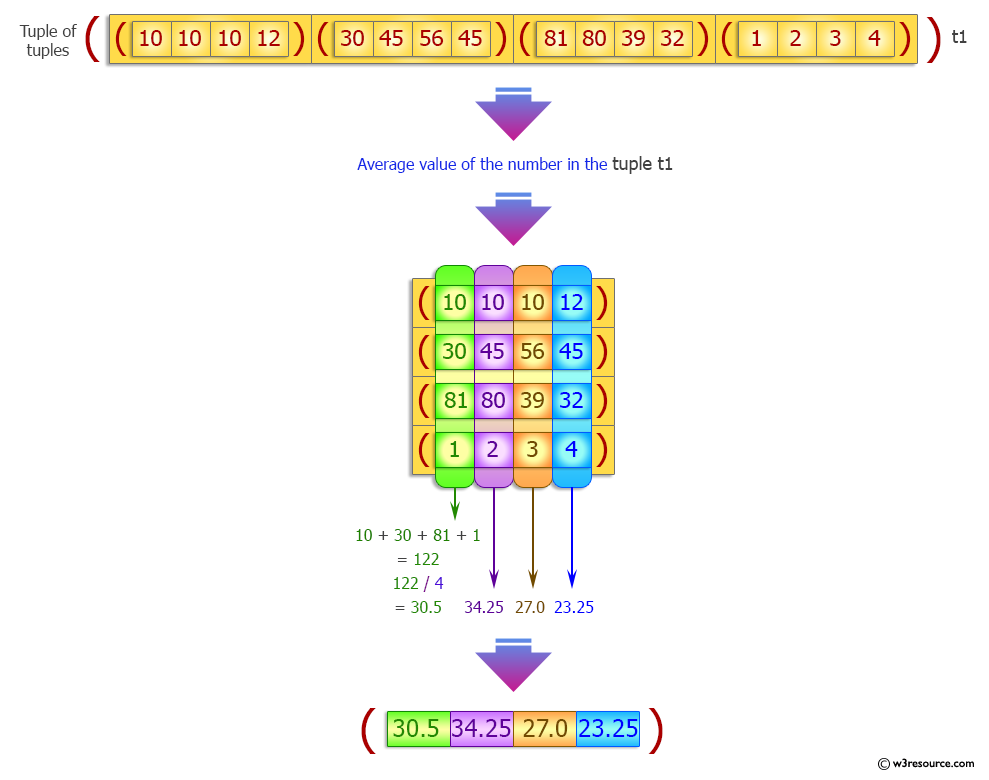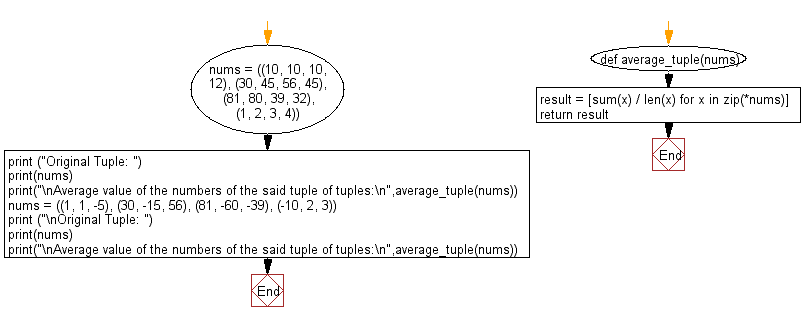﻿ Python: Average value of the numbers in a given tuple of tuples - w3resource# Python Exercise: Average value of the numbers in a given tuple of tuples

## Python tuple: Exercise-27 with Solution

Write a Python program to calculate the average value of the numbers in a given tuple of tuples.

Sample Solution:-

Python Code:

``````def average_tuple(nums):
result = [sum(x) / len(x) for x in zip(*nums)]
return result

nums = ((10, 10, 10, 12), (30, 45, 56, 45), (81, 80, 39, 32), (1, 2, 3, 4))
print ("Original Tuple: ")
print(nums)
print("\nAverage value of the numbers of the said tuple of tuples:\n",average_tuple(nums))

nums = ((1, 1, -5), (30, -15, 56), (81, -60, -39), (-10, 2, 3))
print ("\nOriginal Tuple: ")
print(nums)
print("\nAverage value of the numbers of the said tuple of tuples:\n",average_tuple(nums))
```
```

Sample Output:

```Original Tuple:
((10, 10, 10, 12), (30, 45, 56, 45), (81, 80, 39, 32), (1, 2, 3, 4))

Average value of the numbers of the said tuple of tuples:
[30.5, 34.25, 27.0, 23.25]

Original Tuple:
((1, 1, -5), (30, -15, 56), (81, -60, -39), (-10, 2, 3))

Average value of the numbers of the said tuple of tuples:
[25.5, -18.0, 3.75]
```

Pictorial Presentation:Flowchart:## Visualize Python code execution:

The following tool visualize what the computer is doing step-by-step as it executes the said program:

Python Code Editor:

Have another way to solve this solution? Contribute your code (and comments) through Disqus.

What is the difficulty level of this exercise?

Test your Programming skills with w3resource's quiz.

﻿

## Python: Tips of the Day

Decapitalizes the first letter of a string:

Example:

```def tips_decapitalize(s, upper_rest=False):
return s[:1].lower() + (s[1:].upper() if upper_rest else s[1:])
print(tips_decapitalize('PythonTips'))
print(tips_decapitalize('PythonTips', True))
```

Output:

```pythonTips
pYTHONTIPS
```